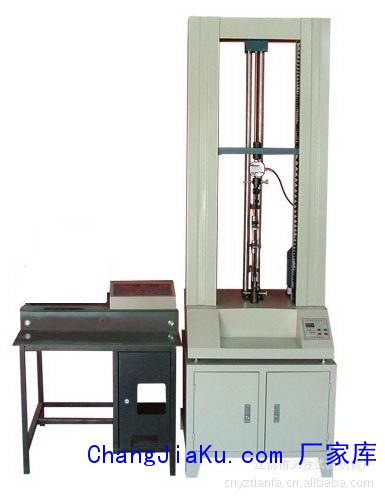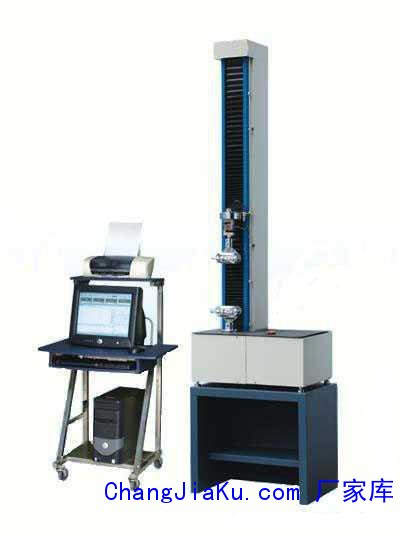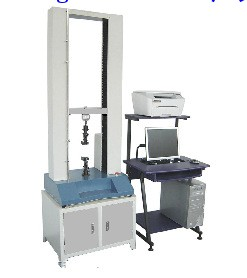客服热线：133-4342-757513852508165•••# 弯曲试验机 剥离试验机 拉伸试验机 材料试验机 【天发老品牌】

• 面议

CJK-1413

13852508165 051486260625
• 发货地  江苏
• 供货总量  100 台

• 暂无产品目录
 有效期至长期有效 更新时间2020-05-09 10:35 访问次数799
r r r r r r r r r r r r r r r r r r r r r r
 r 加工定制:是r r 测力范围:0-5000Nr r 测力精度:0.5%r r 电源:380vr r 外形尺寸:680mm×600mm×1600mm（mm）r r 重量:180（Kg）r r 产品用途:产品适用于金属、非金属、复合材料及制品的拉伸、压缩、弯曲、剪切、撕裂、剥离等物r r 产品规格:0-5000Nr r 拉力机:试验机r
r

r      JDL系列微机控制电子万能试验机（双柱式）r

r

r 特点及用途：JDL系列微机控制电子万能试验机是一种具有国内领先技术的材料检测设备。r

r

r 产品适用于金属、非金属、复合材料及制品的拉伸、压缩、弯曲、剪切、撕裂、剥离等物r

r

r 理性能试验。运用windows98/me/2000/xp操作系统平台，图形图象化的软件界面、灵活的r

r

r 数据处理方式、模块化的VB语言编程方法、安全的限位保护等功能。还具有算法自动生r

r

r 成、试验报告自动编辑功能；大大方便了调试和系统再开发能力，可计算最大力、屈服力、r

r

r 非比例屈服力、平均剥离力、弹性模量等参数；使用高精度的测量仪器，集高度自动化、r

r

r 智能化于一体。其结构新颖，技术先进，性能稳定，操作简单灵活，维护方便，可用于科r

r

r 研部门、大中专院校和工矿企业对各种材料进行力学性能分析和生产质量检验。r

r

r
r

r

r 技术参数：r

r

r
r

r

r 1最大试验力：5KNr

r

r
r

r

r 2、准确度等级：0.5r

r

r
r

r

r 3、负荷测量范围：0.2%—100%FSr

r

r
r

r

r 4、试验力示值允许误差极限：示值的±1%以内r

r

r
r

r

r 5、试验力示值分辨率：最大试验力的1/±300000r

r

r
r

r

r 6、变形测量范围：0.02—100%FSr

r

r
r

r

r 7、变形示值误差极限：示值的±0.50%以内r

r

r
r

r

r 8、变形分辨力：最大变形的1/300000r

r

r
r

r

r 9、位移示值误差极限：示值的±0.5%以内r

r

r
r

r

r 10、位移分辨力：0.05μmr

r

r
r

r

r 11、力控速率调节范围：0.01-10%FS/Sr

r

r
r

r

r 12、力控速率相对误差：±0.5%设置值以内r

r

r
r

r

r 13、变形速率调节范围：0.02—5%FS/Sr

r

r
r

r

r 14、变形控制速率相对误差：±1%设定值以内r

r

r
r

r

r 15、位移速率调节范围：0.001—500mm/minr

r

r
r

r

r 16、位移速率控制精度：速率＜0.01mm/min时，设定值的±1.0%以内r

r

r
r

r

r 速率≥0.01mm/min时，设定值的±0.2%以内r

r

r
r

r

r 17、恒力、恒变形、恒位移控制范围：0.5%—100%FSr

r

r
r

r

r 18、恒力、恒变形、恒位移控制精度：设定值≥10%FS时，r

r

r
r

r

r 设定值的±0.1%以内；设定值＜10%FS时，设定值的±0.5%以内r

r

r
r

r

r 19、有效试验宽度：420mmr

r

r
r

r

r 20、有效拉伸空间距离：900mmr

r

r
r

r

r 21、量程分档：满量程的0.250.500.751.00四档r

r

r
r

r

r 22、行程限位安全装置：电子非接触式限位保护r

r

r
r

r

r 23、超载保护：试验力超过最大试验力10%时机器自动停机r

r

r
r

r

r 24、横梁返回功能：试验结束后横梁以高速返回试验初始位置r

r

r
r

r

r 25、主机外型尺寸（长××高）：680mm×600mm×1600mmr

r

r
r

r

r 26、电源：220V±10%; 50Hzr

r

r 27、主机重量：180kgr

r

r
r

r

r  r

r

r 微机控制电子万能试验机JDL-5000N主要配置r

r

r
r

r

r 一.电子万能试验机概述r

r

r
r

r

r 子万能材料试验机可对橡胶、塑料、塑胶、无纺布、纺织面料、尼龙、纤维、纳米材料、高分子材料、复合材料、包装带、纸张、电线电缆、光纤光缆、安全带、保险带、皮革皮带、鞋类、胶带、聚合物、弹簧钢、轴承钢、不锈钢（及其它高硬度钢）、铸件、钢板、钢带、有色金属、汽车配件、合金材料及其它非金属材料和金属材料进行拉伸、压缩、弯曲、撕裂、90°剥离、180°剥离、剪切、粘合力、拔出力、延伸伸长率等试验。r

r

r
r

r

r 二.电子万能材料试验机主机规格
r
1.量程范围: 0-5000N。
r力量精度在±0.5 以内。
r2.容量分段:全程七档：× 1，× 2，× 5，× 10，× 20，× 50，× 100
r采用高精度24 bits A/D，取样频率200Hz
r全程力量最大解析度 1/1000,000
r3.动力系统:

r4.

r5.数据传输方式：RS232传输
r6.显示方式:UTM107+WIN-XP测试软件计算机屏幕显示。
r7.简洁的全程一档与精密全程七档力量线性双校正系统。
r8.测试界面软件可实现定速度、定位移、定荷重（可设定保持时间）、定荷重增率、定应力增率、定应变增率等控制模式加上多阶控制模式可满足不同的测试要求。
r9.测试空间:测试宽度约450 mm（标准规格）联板行走空间900 mm（不含夹具）（标准规格）
r10.全程位移: 编码器2500 P/R，提升4倍精度采用LINE DRIVE编码器抗干扰能力极强
r位移解析0.001mm。
r11.安全装置:过载紧急停机装置、上下行程限定装置、漏电自动断电系统、自动断点停机功能。
r12．手控方式：可增添无线遥控装置或手动操作盒。（需选购）
r

r

r
r

r

r 三.电子拉力机软件功能介绍:
r
A.测试标准模块化功能:提供使用者设定所需应用的测试标准设定，范围涵盖GB、ASTM、DIN、JIS、BS…等。测试标准规范。
rB. 试品资料:提供使用者设定所有试品数据，一次输入数据永久重复使用。并可自行增修公式以提高测试数据契合性。
rC. 双报表编辑:完全开放式使用者编辑报表，供测试者选择自己喜好的报表格式（测试程序新增内建EXCEL报表编辑功能扩展了以往单一专业报表的格局）
rD. 各长度、力量单位、显示位数采用动态互换方式，力量单位T、Kg、N、KN、g、lb，变形单位mm、cm、inch。
rE. 图形曲线尺度自动最佳化Auto Scale，可使图形以最佳尺度显示。并可于测试中实时图形动态切换。具有荷重-位移、荷重-时间、位移-时间、应力-应变荷重-2点延伸图，以及多曲线对比。
r

r

r
r

r

r
rF
．测试结果可以EXCEL格式的数据形式输出。
rG．测试结束可自动存档、手动存档，测试完毕自动求算最大力量、上、下屈服强度、滞后环法、逐步逼近法、非比例延伸强度、抗拉强度、抗压强度、任意点定伸长强度、任意点定负荷延伸、弹性模量、延伸率、剥离区间最大值、最小值、平均值、净能量、折返能量、总能量、弯曲模量、断点位移x％荷重、断点荷重X％位移、等等。数据备份：测试数据可保存在任意硬盘分区。
rH．多种语言随机切换：简体中文、繁体中文、英文。
rI．软件具有历史测试数据演示功能。
r

r

r
r

r

r 四.电子万能拉力试验机附件
r
A.说明书一份。
rB. 随机配备标准电子万能试验机夹具一组（其他夹具选购）。
rC.电子万能试验机专用测试软件一份。
rD. 清华同方电脑、液晶显示19寸、惠普彩色喷墨打印机。
r

r

r
r

r

r 五.电子拉力试验机可测试项目r

r

r
r

r

r 一）普通测试项目：（普通显示值及计算值）r

r

r
r

r

r 拉伸应力      ●拉伸强度r

r

r
r

r

r 扯断强度      ●扯断伸长率r

r

r
r

r

r 定伸应力      ●定应力伸长率r

r

r
r

r

r 定应力力值    ●撕裂强度r

r

r
r

r

r 任意点力值    ●任意点伸长率r

r

r
r

r

r 抽出力        ●粘合力及取峰值计算值r

r

r
r

r

r 压力试验      ●剪切剥离力试验r

r

r
r

r

r 弯曲试验      ●拔出力穿刺力试验r

r

r
r

r

r 二）特殊测试项目：r

r

r
r

r

r 1.性系数即弹性杨氏模量r

r

r
r

r

r 定义：同相位的法向应力分量与法向应变之比。为测定材料刚性之系数，其值越高，材料越强韧。r

r

r
r

r

r 2.比例限：荷重在一定范围内与伸长可以维持成正比之关系，其最大应力即为比极限。r

r

r
r

r

r 3.弹性限：为材料所能承受而不呈永久变形之最大应力。r

r

r
r

r

r 4.弹性变形：除去荷重后，材料的变形完全消失。r

r

r
r

r

r 5.永久变形：除去荷重后，材料仍残留变形。r

r

r
r

r

r 6.屈服点：材料拉伸时，变形增快而应力不变，此点即为屈服点。屈服点分为上下屈服点，r

r

r
r

r

r 一般以上屈服点作为屈服点。r

r

r
r

r

r 屈服（yield）：荷重超过比例限与伸长不再成正比，荷重会突降，然后在一段时间内，上下起伏，伸长发生较大变化，这种现象叫作屈服。r

r

r
r

r

r 7.屈服强度：拉伸时，永久伸长率达到某一规定值之荷重，除以平行部原断面积，所得之商。r

r

r
r

r

r 8.弹簧K值：与变形同相位的作用力分量与形变之比。r

r

r
r

r

r 9.效弹性和滞后损失：在电子万能材料试验机上，以一定的速度将试样拉伸到一定的伸长率或拉伸到规定的负荷时，测定试样收缩时恢复的功和伸张时消耗的功之比的百分数，即为有效弹性；测定试样伸长、收缩时所损失的能与伸长时所消耗的功之比的百分数，即为滞后损失。r

r

r
r

r

r
r

r

r
r

r

r
r

r

r 扬州市天发试验机械有限公司r

r

r
r

r

r 厂址：江苏省江都市昭关戚运路108r

r

r
r

r

r 电话：0514-862606258626062686260628r

r

r
r

r

r 传真：0514-86260626    邮编：225263r

r

r
r

r

r 联系人：董德发  手机：013905250626r

r

r
r

r

r 网址：www.yztianfa.com      r

r

r
r

r

r 电子邮箱：yztf@yztianfa.comr

r

rr
rr
r
r
rr
r
r

r

r
r

r

r
r

r

r
r

## 拉力试验机 电子试验机 江都天发rJDL系列微机控制电子万能试验机（双柱式）rrr特点及用途：JDL系列微机控制电子万能试验机是一种具有国内领先技术的材料检测设备。产品适用于金属、非金属、复合材料及制品的拉伸、压缩、弯曲、剪切、撕裂、剥离等物理性能试验。运用windows98/me/2000/xp操作系统平台，图形图象化的软件界面、灵活的数据处理方式、模块化的VB语言编程方法、安全的限位保护等功能。还具有算法自动生成、试验报告自动编辑功能；大大方便了调试和系统

## 漆包线试验机、材料试验机、材料拉力试验机## 天发仪器微机屏显液压试验机、液压试验机## JDL伺服拉力试验机、试验机## 供应试验机、拉力试验机（生产厂家，专业制## 金属试验机 拉力试验机、试验机WLB-5000N型机械式拉力机特点与用途：WLB-5000N型拉力试验机为摆锤式、适用于拉力范围0～5000N、速度范围25～500mm/min，**大伸长率为4000%橡胶物理性能试验。它的主要用途是测定橡胶、胶布及其它类似材料的抗张力、伸长率。同时还能研究试样的滞后现象。技术参数：1、拉力范围：外圈：0～1000N**小分度2N中圈：0～2500N**小分度5N内圈：0～5000N**小分度10N2、拉力刻度误差（用挂重法检查）自10%量程起不大于读数的±0.5%3、下夹

## 拉力试验机 试验机 专业制造商rrrr类型:电子拉力试验机rrr测量范围:5（KN）rrrrr测量精度:±0.5rrr**大负荷:5（KN）rrrrr拉伸空间:900（mm）rrr拉伸速度:500（mm/min）rrrrr加工定制:是rrrrrrJDL系列计算机控制电子**试验机rrr特点及用途：JDL系列电子**试验机是一种具有国内**技术的材料检测设备。产品适用于金属、非金属、复合材料及制品的拉伸、压缩、弯曲、剪切、撕裂、剥离等物理性能试验。运用windows98/me/2000/xp操作系统平台，图形图象化的软件界面、

## 厂家直销JDL系列拉力机 电子拉力机## 天发仪器 微控电子试验机（生产厂家）## 厂家供应试验机、拉力试验机（生产厂家，专rrrr类型:电子拉力试验机rrr测量范围:0-5（KN）rrrrr测量精度:示值的±0.5% 以内rrr**大负荷:0-5（KN）rrrrr拉伸空间:900mm（mm）rrr拉伸速度:25mm/min--500mm/min(特殊要求另定）（mm/min）rrrrr加工定制:是rrrrrrrrrrrJDL系列数显电子试验机（单柱式） rrrrrrr特点及用途：JDL系列数显电子**试验机采用微机控制，传感器测力系 rrr统，无级调速。其结构新颖，数字处理准确，操作简单，使用维护方便。 rrr本机是对金属及